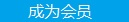您可以捐助，支持我们的公益事业。 1元 10元 50元 认证码：必填求知 文章 文库 Lib 视频 iProcess 课程 角色 咨询 工具 讲座 Modeler Code要资料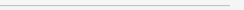订阅捐助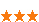377 次浏览     评价： 好 中 差
2019-4-24

 编辑推荐: 本文来自于专知，本文主要详细的对numpy库的操作进行介绍，通过本章节的学习也为之后进行复杂的图像处理打下牢固的基础。

【导读】专知成员Hui上一次为大家介绍Matplotlib的使用，包括绘图，绘制点和线，以及图像的轮廓和直方图，这一次为大家详细讲解Numpy工具包中的各种工具，并且会举实例说明如何应用。Numpy是非常有名的python科学计算工具包，其中包含了大量有用的思想，比如数组对象（用来表示向量、矩阵、图像等等）以及线性代数。Numpy基本操作和图像灰度变换

Python中有好多工具包应用于图像处理当中，本章作为入门章节，首先来介绍Python中最基本的几个工具包，也希望读者可以在之后自行练习。

Numpy

Numpy中的数组对象可以帮助我们进行数组的重要操作，比如矩阵乘积、转置、解方程系统、向量乘积归一化，通过这些操作，我们可以对图像进行建模、图像分类、图像聚类等复杂的图像处理工作。

Numpy可以从www.scipy.org/Download免费下载，在线说明文档(http://docs.scipy.org/doc/numpy/)包含了你可能遇到的大多数问题的答案，供大家参考。

Numpy的基本操作

Numpy在前面的文章中已有涉及，但是并没有系统讲解，今天，我们将详细的对numpy库的操作进行介绍。

 import numpy as np print(np.__version__)

numpy（Numerical Python）提供了python对多维数组对象的支持：ndarray，具有矢量运算能力，快速、节省空间。numpy支持高级大量的维度数组与矩阵运算，此外也针对数组运算提供大量的数学函数库。

 import numpy as np # 引入numpy库 a1 = np.array([1, 2, 3, 4, 5]) # 创建一个一维数组 a2 = np.array([[1, 2, 3, 4, 5], [6, 7, 8, 9, 10]]) # 创建一个二维数组 print('数组a1的长度:', a.shape) # 结果返回一个tuple元组 (2L, 5L) print('数组a2的行数：', a2.shape) # 获得行数，返回 2 print('数组a2的列数：', a2.shape) # 获得列数，返回 5 print('获取矩阵a2的第2行：', a2) # 截取第2行,返回 [ 6 7 8 9 10] print('获取数组a2第一行的第三，四，五个元素：', a2[0, 2:5]) # 截取第二行，第三、四、五列， 返回 [3 4 5] b = a2[a2 > 6] # 截取矩阵a中大于6的元素，范围的是一维数组 print('矩阵中大于6的元素是：', b) # 返回 [ 7 8 9 10] a2[a2 > 6] = 0 print(a2)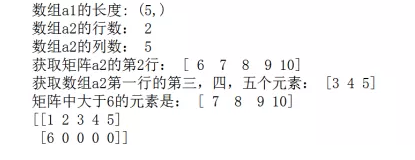a2[a2 % 2 == 1]

 arr[arr % 2 == 1] = -1

 out = np.where(arr % 2 == 1, -1, arr)

np.where 函数是三元表达式 x if condition else y的矢量化版本

 result = np.where(cond,xarr,yarr)

 a1.reshape(2, -1)

 import numpy as np a1 = np.array([[1, 2], [3, 4]]) a2 = np.array([[5, 6], [7, 8]]) # !注意 参数传入时要以列表list或元组tuple的形式传入 print(np.hstack([a1, a2])) # 横向合并 print(np.vstack((a1, a2))) # 纵向合并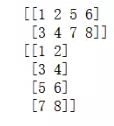np.concatenate( (a1,a2), axis=0 ) 等价于 np.vstack( (a1,a2) ) np.concatenate( (a1,a2), axis=1 ) 等价于 np.hstack( (a1,a2) )

arange函数用于创建等差数组，使用频率非常高，arange非常类似range函数用法为，arange([start,] stop[, step,], dtype=None)根据start与stop指定的范围以及step设定的步长，然后生成对象，例如：

 a2 = np.arange(5,20,2) # 从5开始到20（不包括20），步长为2

a2 = np.arange(5,20,2) # 从5开始到20（不包括20），步长为2

linspace()和matlab的linspace很类似，用于创建指定数量等间隔的序列，实际生成一个等差数列，例如：

 a = np.linspace(0,10,7) # 生成首位是0，末位是10，含7个数的等差数列

a = np.linspace(0,10,7) # 生成首位是0，末位是10，含7个数的等差数列

linspace用于生成等差数列，而logspace用于生成等比数列，例如：

 a = np.logspace(0,2,5)

ones创建全1矩阵

zeros创建全0矩阵

eye创建单位矩阵

empty创建空矩阵（实际有值）

 import numpy as np a_ones = np.ones((3, 4)) # 创建3*4的全1矩阵 print('第一个矩阵：\n', a_ones) a_zeros = np.zeros((3, 4)) # 创建3*4的全0矩阵 print('第二个矩阵：\n', a_zeros) a_eye = np.eye(3) # 创建3阶单位矩阵 print('第三个矩阵：\n', a_eye) a_empty = np.empty((3, 4)) # 创建3*4的空矩阵 print('第四个矩阵：\n', a_empty)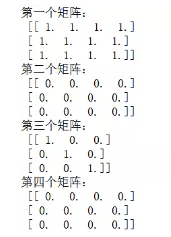+：矩阵对应元素相加 -：矩阵对应元素相减 *：矩阵对应元素相乘 /：矩阵对应元素相除，如果都是整数则取商 %：矩阵对应元素相除后取余数 **：矩阵每个元素都取n次方，如**2：每个元素都取平方

 np.sin(a)：对矩阵a中每个元素取正弦,sin(x) np.cos(a)：对矩阵a中每个元素取余弦,cos(x) np.tan(a)：对矩阵a中每个元素取正切,tan(x) np.arcsin(a)：对矩阵a中每个元素取反正弦,arcsin(x) np.arccos(a)：对矩阵a中每个元素取反余弦,arccos(x) np.arctan(a)：对矩阵a中每个元素取反正切,arctan(x) np.exp(a)：对矩阵a中每个元素取指数函数,ex np.sqrt(a)：对矩阵a中每个元素开根号√x

 import numpy as np a1 = np.array([[1, 2, 3], [4, 5, 6]]) # a1为2*3矩阵 a2 = np.array([[1, 2], [3, 4], [5, 6]]) # a2为3*2矩阵 print(a1.shape == a2.shape) # 用来判断矩阵是否满足点乘的条件 print(a1.dot(a2))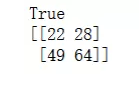import numpy as np import numpy.linalg as lg a = np.array([[1, 2, 3], [4, 5, 6], [7, 8, 9]]) print(lg.inv(a))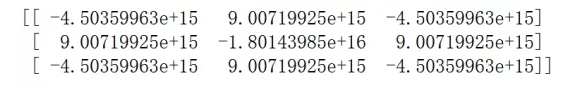im = array(Image.open('test.jpg')) print(im.shape) print(im.dtype)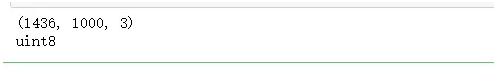im = array(Image.open('test.jpg').convert('L'),'f') print(im.shape) print(im.dtype)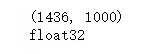from PIL import Image from numpy import * image = array(Image.open('test.jpg').convert('L')) fig = plt.figure(figsize=(30, 30)) plt.subplot(4, 1, 1) plt.imshow(image, cmap=cm.gray) plt.axis("off") plt.subplot(4, 1, 2) image1 = 255 - image plt.imshow(image1, cmap=cm.gray) plt.axis("off") plt.subplot(4, 1, 3) imgae2 = (image / 255.0) * 100 + 100 data2 = np.array(image2) plt.imshow(data2, cmap=cm.gray) plt.axis("off") plt.subplot(4, 1, 4) imgae3 = 255.0 * (image / 255.0) ** 2 data3 = np.array(image3) plt.imshow(data3, cmap=cm.gray) plt.axis("off")

plt.show()输出图像如下：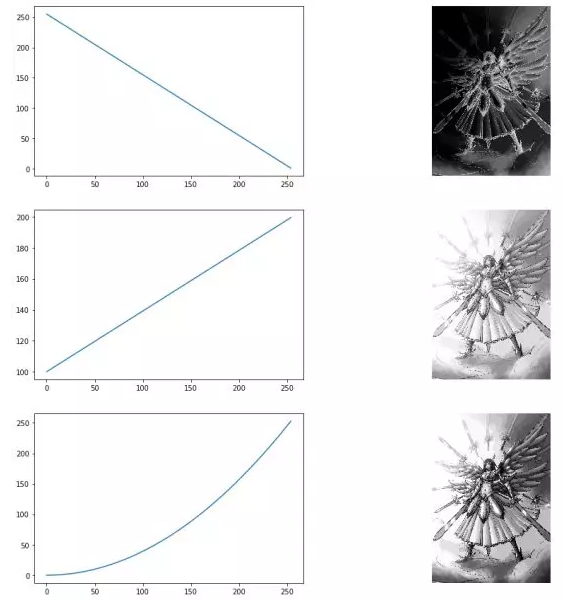377 次浏览  评价: 好 中 差订阅捐助
 相关文章 我们该如何设计数据库 数据库设计经验谈 数据库设计过程 数据库编程总结
 相关文档 数据库性能调优技巧 数据库性能调整 数据库性能优化讲座 数据库系统性能调优系列
 相关课程 高性能数据库设计与优化 高级数据库架构师 数据仓库和数据挖掘技术 Hadoop原理、部署与性能调优每天2个文档/视频 扫描微信二维码订阅
 订阅技术月刊 获得每月300个技术资源